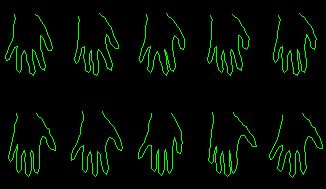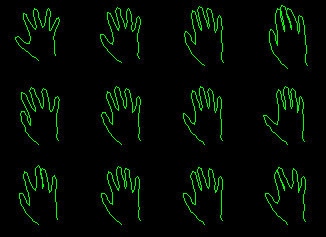# Statistical Shape Models

Given a set of examples of a shape, we can build a statistical shape model. Each shape in the training set is represented by a set of n labelled landmark points, which must be consistant from one shape to the next. For instance, on a hand example, the 7th point may always correspond to the tip of the thumb.

Given a set of such labelled training examples, we align them into a common co-ordinate frame using Procrustes Analysis. This translates, rotates and scales each training shape so as to minimise the sum of squared distances to the mean of the set.

Each shape can then be represented by a 2n element vector

x = (x_1, ... , x_n, y_1, ... , y_n).

The aligned training set forms a cloud in the 2n dimensional space, and can be considered to be a sample from a probability density function.

In the simplest formulation, we approximate the cloud with a gaussian. We use Principal Component Analysis (PCA) to pick out the main axes of the cloud, and model only the first few, which account for the majority of the variation.

The shape model is then

x = x_mean + Pb

where x_mean is the mean of the aligned training examples, P is a 2n x t matrix whose columns are unit vectors along the principal axes of the cloud, and b is a t element vector of shape parameters.

(This model has been dubbed a "Point Distribution Model" (PDM), but has little to do with the Point Distribution in statistics)

By varying the shape parameters within limits learnt from the training set, we can generate new examples.

Such models are used in the Active Shape Model framework to locate new examples in new images.

## Hand Example

Consider the outline of a hand, represented by 72 labelled points.

Here are some examples from a training set:By varying the first three parameters of the shape vector, b, one at a time, we can demonstrate some of the modes of variation allowed by the model:(Each row obtained by varying on parameter and fixing others at zero)

## More Complex Shape Models

In some situations, the gaussian approximation is simplistic, as the pdf for the shapes is significantly non-gaussian. This can arise if sub-parts of the modelled object rotate, leading to curved clouds of training points in the shape space.

Several methods have been used to model such clouds. Polynomial approximations (Sozou et al), neural-net formulations (Sozou et al), and polar co-ordinates (Heap & Hogg) have all been tried. The most recent approach is to treat the cloud as a sample from a pdf, use kernel methods to estimate the pdf, and then to approximate this pdf using a mixture of gaussians. The Expectation Maximisation algorithm is used to fit the mixture to the data. To simplify the calculations, PCA is used to determine a low dimensional sub-space containing most of the variation, before the kernel method is applied. In effect, we use the same model as above, but determine stricter limits on the allowed values of the shape parameters b, modelling their distribution with a mixture of gaussians instead of a single gaussian. The allowed values are those for which the pdf is above a threshold, which can be determined using monte-carlo methods from the mixture.

Tim Cootes